#Function Repository Resource:

# IsingModelCA

Simulate the Ising model using a cellular automata

Contributed by: Stephen Wolfram
 ResourceFunction["IsingModelCA"][n,percent,k] gives the kth step in the evolution of an n×n Ising model with a percentage percent of black cells. ResourceFunction["IsingModelCA"][n,percent,k,j] gives the jth slice in the evolution of an Ising model.

## Details and Options

The Ising model is an idealization of ferromagnetic materials involving an n×n array of spins, each pair of adjacent spins contributes -1 or +1 to the total energy, depending on whether they are parallel or anti-parallel. When the temperature decreases past a critical value, a phase transition occurs between the disordered state and one in which spins tend to align spontaneously, producing magnetization even in the absence of an external field. Technically, in the two-dimensional Ising model, a phase transition only occurs in the limit n→∞.
In physical terms, the value of percent defines the effective temperature (relative to the spin-coupling interaction strength) of the model.

## Examples

### Basic Examples

Evolution with 50% of black cells:

 In:=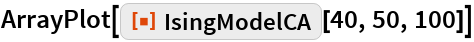Out=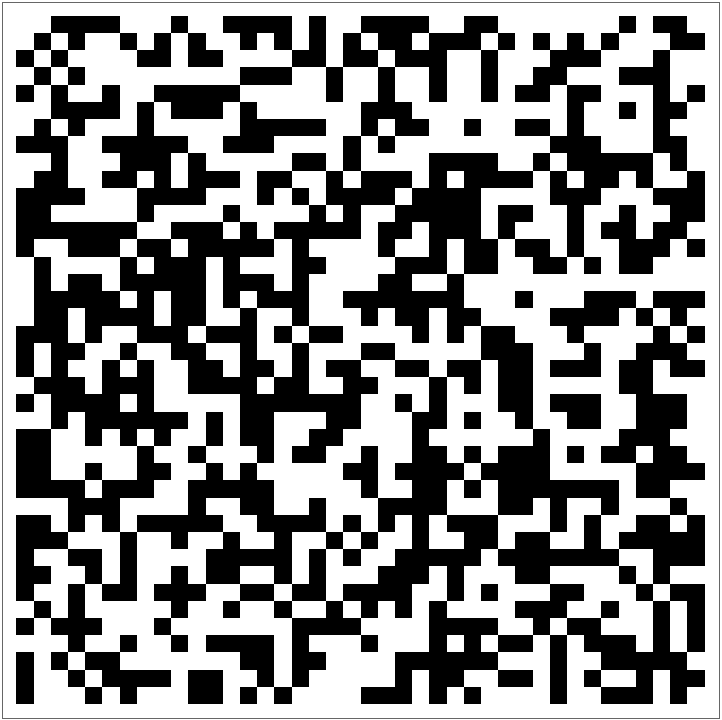Configurations generated after 50 steps from various initial densities:

 In:=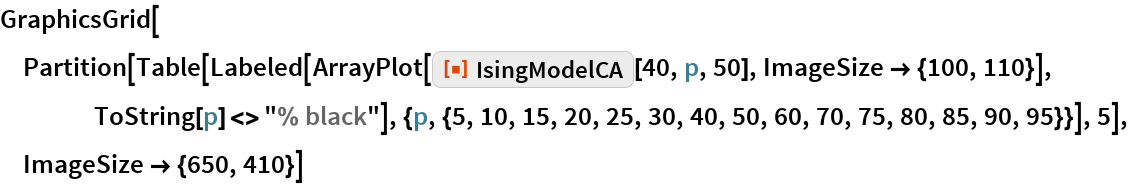Out=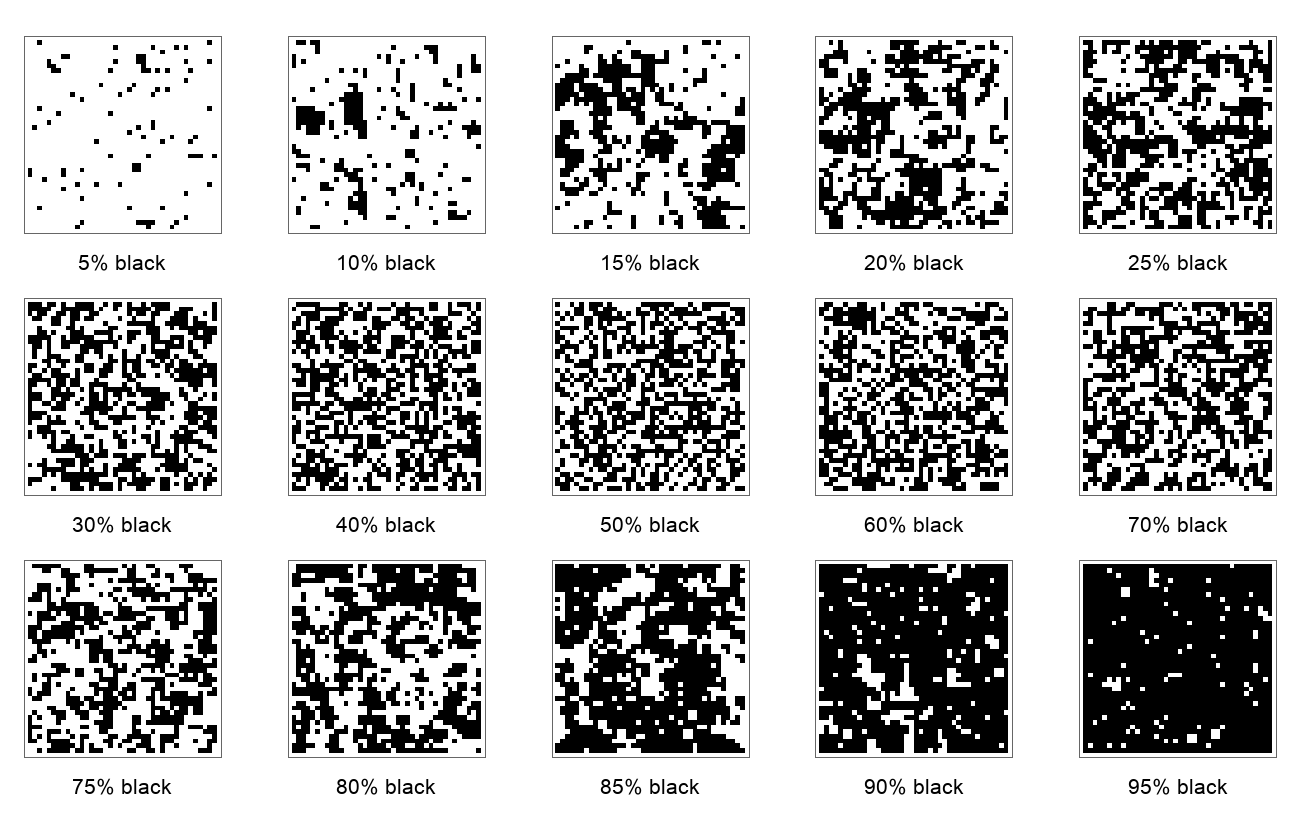Slices through their evolution:

 In:=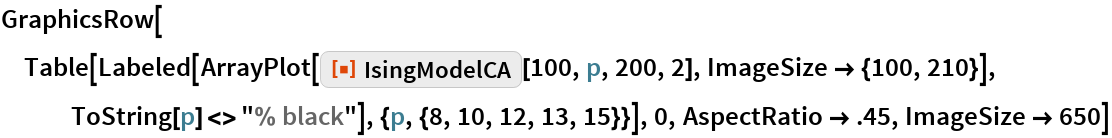Out=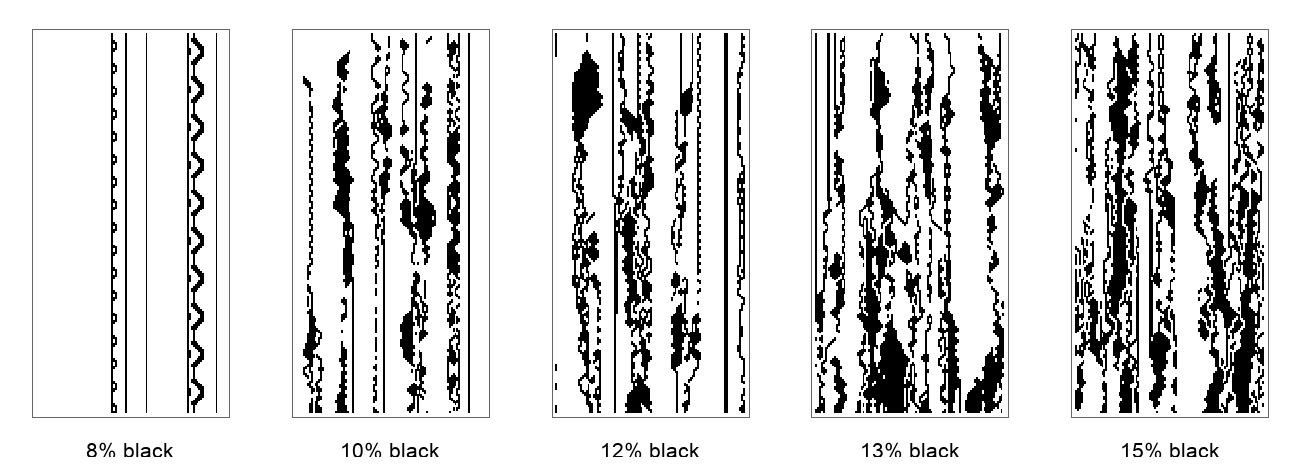## Requirements

Wolfram Language 11.3 (March 2018) or above